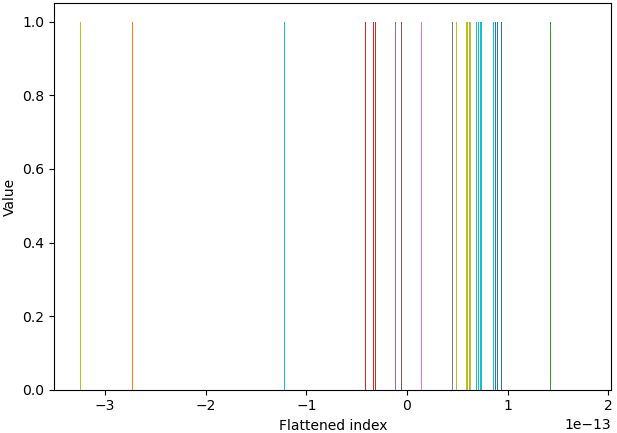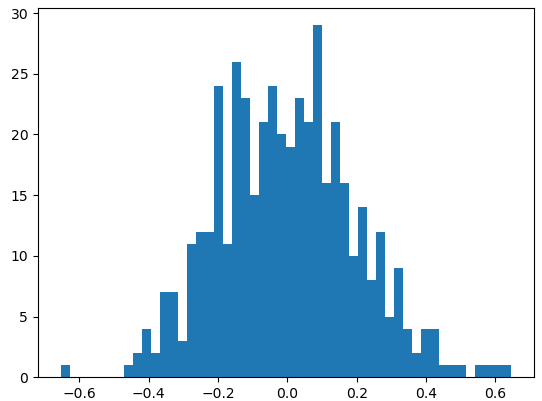# [Bug for 3.3.2] Histogram for small decimal numbers

I am trying to use `ax.hist(vector1d, 20, density=0)` to plot the histogram, where the vector1d is a tensor of decimals in the level of 1e-13, i.e.,

``````>>> one_batch_error_grad
tensor([-1.3246e-13, -1.0851e-13, -1.0851e-13,  ...,  5.2838e-14,
4.4859e-14, -3.1314e-14])
``````

But I got the histogram as below, since like the `ax.hist()` cannot deal with small decimal numbers? I have used it forIs it because of the version? I am using matplotlib==3.3.2

1 Like

I just tried a more simple tensor with matplotlib==3.2.2, the `ax.hist()` result is as below:While the same code is not well with matplotlib==3.3.2:1 Like

Please make a reproducible example otherwise it’s hard to help.